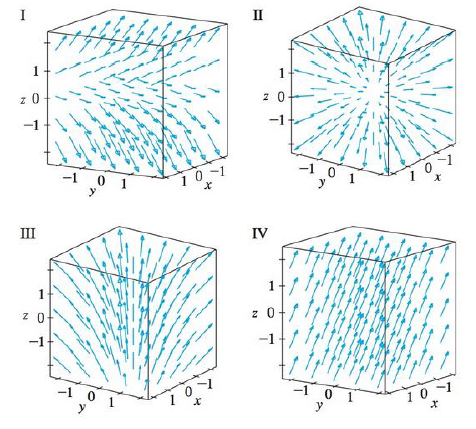Chapter 16.1, Problem 17E

Chapter
Section
Textbook Problem

Match the vector fields F on ℝ3 with the plots labeled I-IV. Give reasons for your choices.17. F(x, y, z) = x i + y j + 3 kTo determine

To match: The vector field F(x,y,z)=xi+yj+3k with the plots labeled as I-IV.

Explanation

Given data:

F(x,y,z)=xi+yj+3k=x,y,3

Formula used:

Consider a three-dimensional vector F=x,y,z .

Write the expression for length of the three dimensional vector.

|F(x,y,z)|=x2+y2+z2 (1)

Find the length of F(x,y,z) using equation (1)

Still sussing out bartleby?

Check out a sample textbook solution.

See a sample solution

The Solution to Your Study Problems

Bartleby provides explanations to thousands of textbook problems written by our experts, many with advanced degrees!

Get Started

In Exercises 1-6, simplify the expression. 3+2x7x

Calculus: An Applied Approach (MindTap Course List)

In Exercises 69-74, rationalize the numerator. 69. 2x3

Applied Calculus for the Managerial, Life, and Social Sciences: A Brief Approach

In problems 63-73, factor each expression completely. 66.

Mathematical Applications for the Management, Life, and Social Sciences

The arc length function for y = x2, 1 x 3 is s(x) = a) 1x1+4x2dx b) 1x1+4t2dt c) 1x1+t4dt d) 1x1+4t4dt

Study Guide for Stewart's Single Variable Calculus: Early Transcendentals, 8th

Explain how a researcher using simple random sampling can still obtain a biased sample.

Research Methods for the Behavioral Sciences (MindTap Course List)